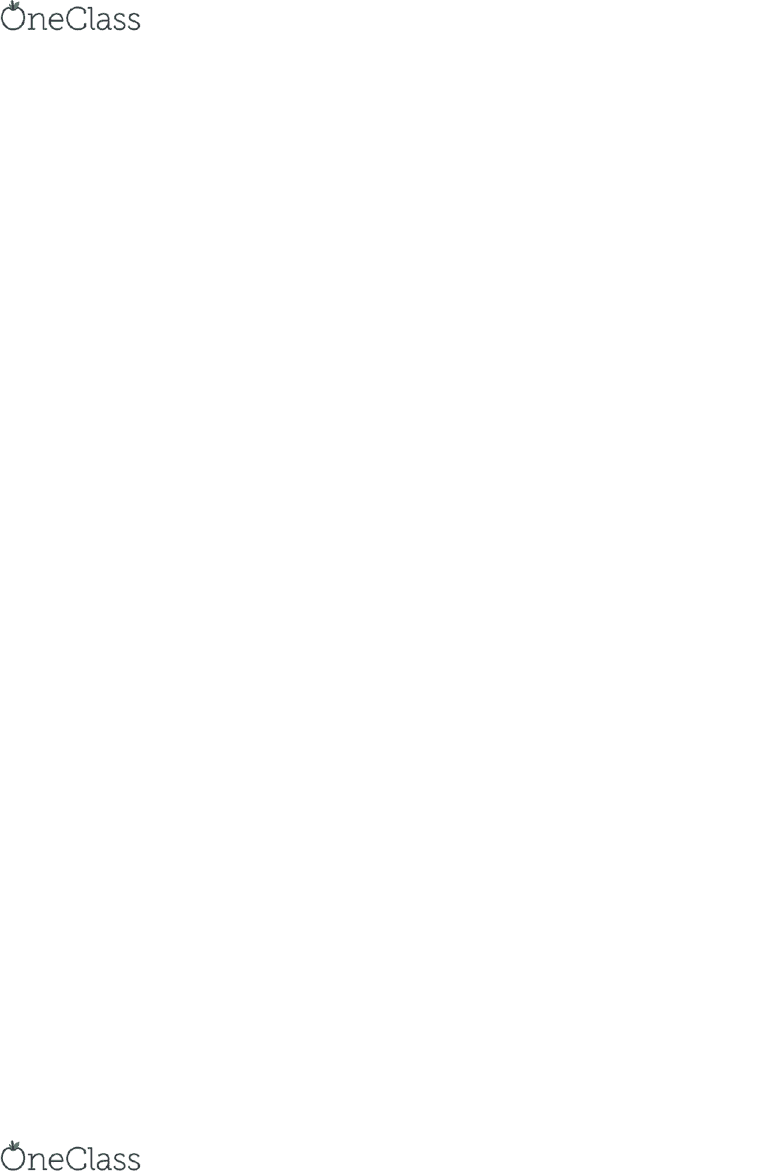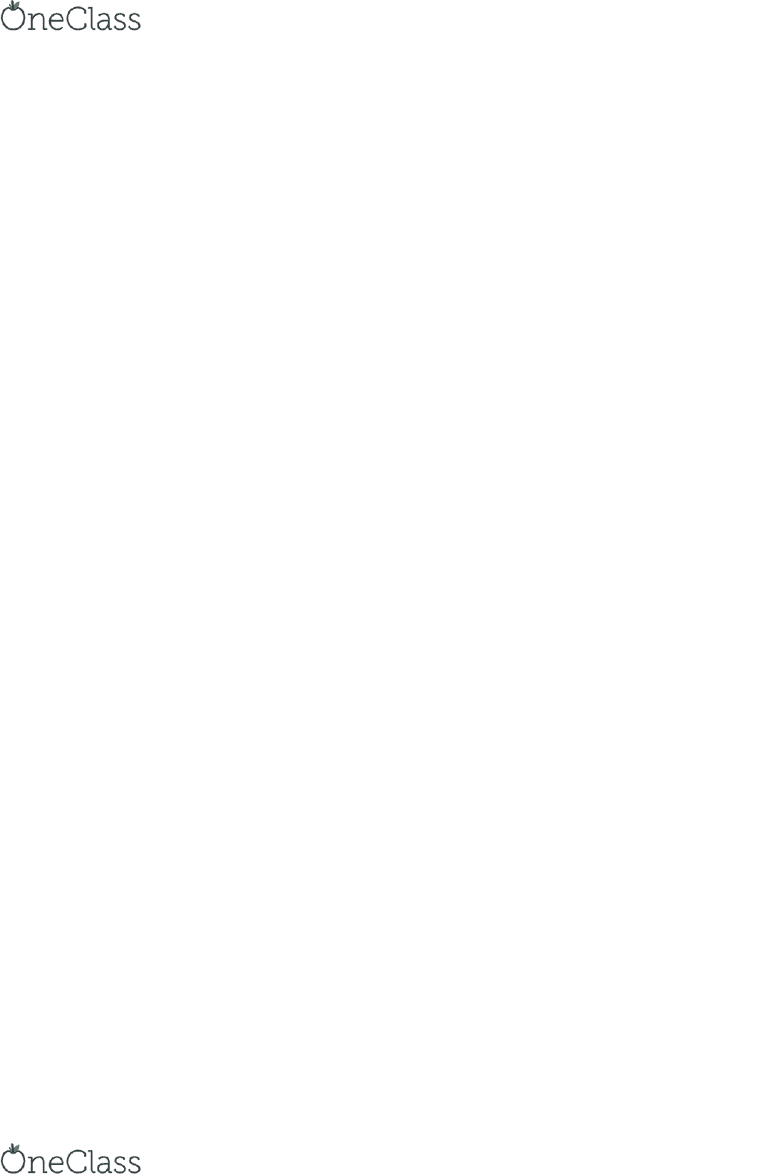Class Notes (1,100,000)
US (480,000)
TAMU (6,000)
AGEC (10)
Lecture 9

# AGEC 330 Lecture Notes - Lecture 9: Tax Rate, Center Pivot Irrigation, Cash Flow Statement

Department
Agricultural Economics
Course Code
AGEC 330
Professor
David Leatham
Lecture
9

This preview shows pages 1-3. to view the full 21 pages of the document.A farmer is considering borrowing money from a bank. Given the following information: 5/10
Initial loan amount is \$52,000.
The loan will be fully amortized in 3 years at 10%.
Marginal tax rate is 15%.
i) What is the loan balance at the end of 1st year? ~ \$36,290.03
ii) What is the loan balance at the end of 2nd year?~ \$23,957.70
A farmer is considering borrowing money from a bank. Given the following information:
Initial loan amount is \$250,000.
The loan will be fully amortized in 3 years at 14%.
Marginal tax rate is 15%.
What is the principal payment in the 1st year?~ \$72,682.87
What is the principal payment in the 2nd year?~ \$82,858.47
A farmer is considering borrowing money from a bank. Given the following information: 5/10
Initial loan amount is \$250,000.
The loan will be fully amortized in 3 years at 14%.
Marginal tax rate is 15%.
What is the tax saving in the 1st year?~ \$6,176.47
What is the tax saving in the 2nd year?~ \$3,723.66
Principal payments on a fully amortized loan decrease over the life of the loan. FALSE
Tax Savings from interest can be found by multiplying the before-tax risk free discount rate by the
interest paid. FALSE
______________ is the s um of principal payme nt and inte rest payme nt.
Loa n P ayment pe r period
Fully Amortized loa n implie s a n e qual periodic pa yment including principa l a nd intere st.
TRUE
A fa rmer is conside ring borrowing mone y from a ba nk. Give n the following informa tion:
Initial loan a mount is \$66,000.
The loa n will be fully a mortized in 3 yea rs a t 8%.
Ma rgina l ta x ra te is 15%.
A~ \$25,610.21
A rancher borrowe d money to purcha se a new truck 4 yea rs ago. The annual loan
payme nt is \$32,000, the interes t rate is 4% a nd there are 2 yea rs le ft on the loa n. How
much mone y does the ra ncher s till owe on the loa n?
~ \$60,355.03
Given the following loa n informa tion:
Annua l loa n payme nt = \$18,000
Numbe r of rema ining pe riods = 4
Inte res t rate = 10%
Wha t is the book va lue of the loan?
find more resources at oneclass.com
find more resources at oneclass.com

Only pages 1-3 are available for preview. Some parts have been intentionally blurred.~ \$57,057.58
Given the following informa tion:
Initial Loa n Amount = \$36,200
Inte re st Ra te = 6%
Fully a mortize d loa n over 10 ye a rs .
What is the annual loan payment?
~ \$4,918.42
What is the remaining principal balance on the following loan at the end of 4 years?
~ \$24,185.47
A farmer wants to borrow \$97,300 for a tractor. The loan would be fully amortized over 20 years
at 14% interest.
Annual loan payment~ 15,841.31 remaining prin balance 15 years ~ 50,435.18
Projected Cash Flow Statement can be used to determine if an investment is financially
feasible.~ TRUE
Cash flows stated in nominal dollars over time cannot be converted to real dollars. ~ FALSE
Infla tion is a n increa s e in the ge ne ral le ve l of price s for a ll goods a nd se rvices in a n
economy. ~TRUE
Rea l Price s a re the prices that reflect today’s purchasing power at a specific point in
time . ~TRUE
What is the nomina l price of a truck in 15 ye a rs if the re a l price is \$25,000, a nd the
infla tion rate is 3%? ~\$38,949.19
The yield on a bond is 15% and infla tion is expected to be 5%. Ca lcula te the re a l
inte re s t ra te on the bond. ~9.52%
_____________ is a n a mount that ma kes you indiffe re nt betwe e n playing a nd not
pla ying the game . ~ Certa inty e quivalent
Ris k premium doe s not de pend on : individua ls budget
Given the following information :
Nominal Initial Cost = \$30,000; Nominal Before-tax Net Return = \$4,000
Marginal Tax Rate = 15%; Required rate of return = 10%
Real Terminal Value = \$20,000; Investment Life = 8 years
Suppose that IRS will allow the investor to depreciate the investment using straight-line over 10 years and the inflation
rate is 4%.
find more resources at oneclass.com
find more resources at oneclass.com

Only pages 1-3 are available for preview. Some parts have been intentionally blurred.(i) What is the annual depreciation expense?
a. \$3,450 b. \$3,750
c. \$3,120 d. \$3,000
enter response here: [i]
(ii) What are the tax savings from depreciation?
a. \$450 b. \$300
c. \$120 d. \$600
enter response here: [ii]
d
a
A farmer expects irrigation system will increase real operating receipts by \$20,000 per year but will also increase real
operating expenses by \$8,000. Suppose that the inflation rate is 4% and the marginal tax rate is 20%.
(i) What is the nominal net return at the end of year 2?
a. \$12,000 b. \$14,400
c. \$12,972 d. \$11,520
ENTER RESPONSE HERE: [i]
(ii) Calculate the nominal after-tax net return at the end of year 2.
a. \$10,383 b. \$13,824
c. \$14,400 d. \$17,280
ENTER RESPONSE HERE: [ii]
c
a
Nominal cash flows can be discounted by either a nominal discount rate or a real discount
rate.
False
Nominal cash flows can be discounted by either a nominal discount rate or a real discount
rate. However, real cash flows must be discounted by a real discount rate only.
False
Real cash flows can be discounted by a real discount rate only.
True
Suppose that the inflation rate is 4% and the real terminal value of an investment is
expected to be \$70,000 in 4 years. Calculate the nominal terminal value of the investment
at the end of year 4.
\$81,890
Given the following information:
Nominal Initial Cost = \$25,000; Nominal Before-tax Net Return = \$5,000
Marginal Tax Rate = 15%; Required rate of return = 10%
find more resources at oneclass.com
find more resources at oneclass.com